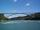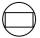Chord 3

What is the radius of the circle where the chord is 2/3 of the radius from the center and has a length of 10 cm?

Result

r =  6.71 cm

Solution:Leave us a comment of example and its solution (i.e. if it is still somewhat unclear...):Be the first to comment!To solve this example are needed these knowledge from mathematics:

Pythagorean theorem is the base for the right triangle calculator.

Next similar examples:

1. Common chordTwo circles with radius 17 cm and 20 cm are intersect at two points. Its common chord is long 27 cm. What is the distance of the centers of these circles?
2. Concentric circlesIn the circle with diameter 19 cm is constructed chord 9 cm long. Calculate the radius of a concentric circle that touches this chord.
3. The chordCalculate a chord length which the distance from the center of the circle (S, 6 cm) equals 3 cm.
4. Chord distanceThe circle k (S, 6 cm), calculate the chord distance from the center circle S when the length of the chord is t = 10 cm.
5. Chord 5It is given circle k / S; 5 cm /. Its chord MN is 3 cm away from the center of the circle . Calculate its length.
6. Chord circleThe circle to the (S, r = 8 cm) are different points A, B connected segment /AB/ = 12 cm. AB mark the middle of S'. Calculate |SS'|. Make the sketch.
7. CancerOf the 80 people 50 people ill cancer. What percentage of people isn't ill?
8. Two circlesTwo circles with a radius 4 cm and 3 cm have a center distance 0.5cm. How many common points have these circles?
9. BridgeThe bridge arc has a span 235 m and height 3 m. Calculate the radius of the circle arc of this bridge.
10. AceThe length of segment AB is 24 cm and the point M and N divided it into thirds. Calculate the circumference and area of this shape.
11. Rectangle and circleThe rectangle ABCD has side lengths a = 40 mm and b = 30 mm and is circumscribed by a circle k. Calculate approximately how many cm is circle long.
12. Circular lawnAround a circular lawn area is 2 m wide sidewalk. The outer edge of the sidewalk is curb whose width is 2 m. Curbstone and the inner side of the sidewalk together form a concentric circles. Calculate the area of the circular lawn and the result round to 1
13. WindbreakA tree at a height of 3 meters broke in the windbreak. Its peak fell 4.5 m from the tree. How tall was the tree?
14. Broken treeThe tree was 35 meters high. The tree broke at a height of 10 m above the ground. Top but does not fall off it refuted on the ground. How far from the base of the tree lay its peak?
15. Four ropesTV transmitter is anchored at a height of 44 meters by four ropes. Each rope is attached at a distance of 55 meters from the heel of the TV transmitter. Calculate how many meters of rope were used in the construction of the transmitter. At each attachment.
16. Waiting roomIn the waiting room are people and flies. Together they have 15 heads and 50 legs (fly has 6 legs). How many people and flies are in the waiting room?
17. CandyPeter had a sachet of candy. He wanted to share with his friends. If he gave them 30 candies, he would have 62 candies. If he gave them 40 candies, he would miss 8 candies. How many friends did Peter have?